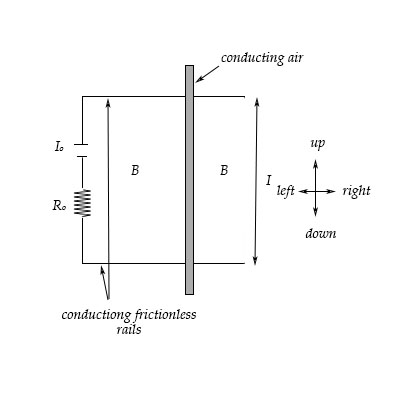# Consider a battery emf = V_o attached to two conducting frictionless rails that are a distance l...

## Question:

Consider a battery {eq}emf = V_o {/eq} attached to two conducting frictionless rails that are a distance l apart. There is magnetic field {eq}B {/eq} perpendicular to them as well as the field, as shown in the diagram below (Note, assume that any field effects from the battery are negligible.) The bar is placed on the rails, starts from rest, and accelerates conducting bar left down conducting friction less rails

Consider the situation as described and select True or False for each of the following statements:

a) The direction of the induced e.m.f in the bar will be up

b) The bar will accelerate away from the battery

c) Faraday's Law is such that changing magnetic fields generate electric fields

d) If the magnetic field were reversed, the bar would accelerate towards the battery

e) Lenz's Law is such that induced currents produce magnetic fields that tend to oppose the flux changes that induce those currents.One of the most interesting laws of electromagnetism is Faraday's Law of induction. Faraday conducted several experiments to probe the electromagnetic induction.

• If we take two solenoids and connect one to a battery and a switch and the other one to a very sensitive galvanometer when we close and open the circuit, during a small interval of time we detect a small current in the galvanometer.
• If we take a magnet, and we move the magnet forward and toward a closed-loop, also connected to a solenoid, while the magnet is moving we will detect a current in the galvanometer.

In both cases, the sign of the current will be different if we are closing or opening the circuit, or if the magnet is getting close to the loop or getting far.

Faraday's Law in mathematical form is written as,

{eq}\displaystyle \oint\vec{E}\cdot\vec{dl}=-\dfrac{d \phi_B}{d t} {/eq}.

This means that the circulation of the induced electric field equals the change of the flux of the magnetic field.

In the figure it is not clear in which direction is the magnetic field, in our answer, we will assume it is directed entering the plane of the figure. Due to the polarity of the battery, once the bar is placed on the rails, a current starts to flow going down in the bar. Therefore a magnetic force acts on the bar,

{eq}\vec{F}_m=I_0\vec{L}\times \vec{B} {/eq},

where {eq}I_0 {/eq} is the current in the loop due to the battery and {eq}\vec{L} {/eq} is a vector pointing down.

Applying the rules of the cross product, the magnetic force on the bar is directed to the right.

Therefore the bar starts to accelerate to the right. As a consequence the flux of the magnetic field in the loop formed by the rails and the bar increases. Lenz's Law establishes that the induced electromotive force counteracts the effect that produces it. If the flux of the magnetic field is increasing in one direction, we need a flux in the opposite direction, in this case, pointing forward from the plane of the figure. To obtain this magnetic field, we need an induced current in the bar going up, which using the right-hand rule, gives the desired magnetic field. Hence the direction of the induced e.m.f. in the bar is up.

a) This statement is {eq}\boxed{TRUE} {/eq}.

b) This statement is {eq}\boxed{TRUE} {/eq}.

Argumentations to both answers are given in the text above.

c) This statement is{eq}\boxed{TRUE} {/eq}.

From the mathematical point of view, Faraday's Law states, written in differential form,

{eq}\nabla\times \vec{E}=\dfrac{\partial \vec{B}}{\partial t} {/eq}.

This means that a time-dependent magnetic field generates an irrotational electric field. This law combined with Ampere-Maxwell's Law explains the existence of electromagnetic waves.

d) This statement is{eq}\boxed{TRUE} {/eq}.

If we reverse the magnetic field, the magnetic force is now to the left, and the bar starts to accelerate towards the battery.

e) This statement is{eq}\boxed{TRUE} {/eq}. This was explained at the beginning of the answer where we enunciate Lenz's Law.